# Thermal Design

As you have probably noticed, my knowledge base articles are free of advertising. Instead of distracting you with annoying ads, I kindly request your donation. If you find the contents of this page to be useful, please consider making a donation by clicking the Donate button below.Thermal Design: Section Outline

A sticking point for many DIY enthusiasts appears to be thermal design. This is rather unfortunate, as thermal design is very important in chip amp applications. The math is as simple as Ohm's Law, E = I × R, so it appears the challenge is mainly related to the terminology used in thermal design.

For reasons that will become obvious in a bit, an analogy between the thermal domain and the electrical domain can be made. This analogy allows for the use of "electrical equations", such as Ohm's Law, on thermal systems. The relevant bits of the analogy are tabulated below.

Thermal Property Symbol Thermal Unit Electrical Analogy Symbol Electrical Unit
Power P W Current I A
Thermal Resistance θ W/°K = W/°C Electrical Resistance R Ω
Temperature T °C Voltage E V

Note: All equations on this page use SI units except where noted. If in doubt, assume SI units.

The biggest challenge in thermal design is often to obtain the thermal resistances needed for the calculations, in particular the thermal resistance of the heat sink. If not provided by the manufacturer, I know of two ways to obtain this data:

1. Mount a power transistor or power resistor to the heat sink, connect it to a power supply, and operate the circuit at a known power dissipation until the heat sink temperature has fully stabilized. Measure the temperature of the ambient air and the heat sink. The thermal resistance can then be calculated as: θsa = (Theat sink - Tambient)/Pdiss.
2. Find an extrusion in Aavid's catalog that matches your heat sink and use their thermal resistance simulator to calculate its thermal resistance. Aavid: North American Extrusions; European Extrusions. If an exact match is not found, it is usually possible to find an extrusion that is reasonably close in terms of width, breadth, length, fin dimensions, and base thickness.

Temperature & Length Correction

Note that heat sink manufacturers tend to specify the thermal resistance of their extrusions for a 3" (76.2 mm) long section of extrusion operating 75 °C above ambient temperature. If you operate your heat sink at a different temperature differential or use a length different than 3", you will need to apply a couple of correction factors.

The thermal performance is measured at a temperature differential of 75 °C, i.e. the heat sink temperature is 75 °C above ambient temperature. Unless your are planning to operate the LM3886 near or above its thermal limit, you will likely have to reduce the temperature differential. Sadly, this means the heat sink becomes less efficient. At a temperature differential of 30 °C, the heat sink has a 25.7 % higher effective thermal resistance (Aavid, temperature correction factors), hence, multiply the specified thermal resistance by 1.257 to obtain the effective thermal resistance at ΔT = 30 °C.

A longer heat sink of a given extrusion profile will have a lower thermal resistance (heat up less for a given power dissipation) than a shorter length of the same extrusion. Unfortunately, the relationship is far from linear. Again, Aavid comes to the rescue with a table of length correction factors. For example, a 6" piece of an extrusion specified for a thermal resistance of 1.0 °C/W at 3", ΔT = 75 °C will have a thermal resistance of 1.0 * 0.73 = 0.73 °C/W at ΔT = 75 °C.

If both the temperature differential and length are different from the specified values, the two correction factors multiply. If our example heat sink extrusion (1.0 °C/W @ 3", ΔT = 75 °C) is cut to 6" and operated at ΔT = 30 °C, the math works out as follows: 1.0 * 0.73 * 1.257 = 0.92 °C/W @ 6", ΔT = 30 °C.

Thermal Design Example 1: The Engineering Solution

A well engineered amplifier should be able to handle anything the user throws at it, including sine wave testing at the highest power dissipation. Hence, the thermal design originates from the power dissipated in the LM3886. This can be calculated, but the math gets a bit hairy. Thankfully, National was kind enough to provide graphs for 4 Ω and 8 Ω loads in the data sheet (Figures 35 and 36). The power dissipated in the LM3886 versus output power and supply voltage is shown below for a 4 Ω load.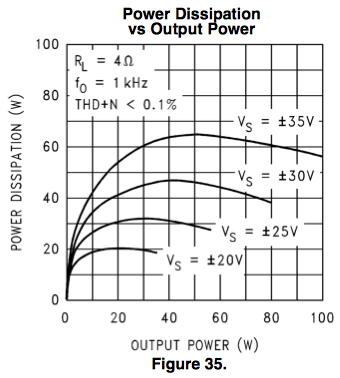For this example, let's assume a safe and sane supply voltage of ±25 V. From the graph, the peak power dissipated in the LM3886 is found to be about 32 W.

For the ambient temperature, I normally use 25 °C (just shy of 80 °F). This jives pretty well with the temperature in a warm living room.

The maximum allowable heat sink temperature is the subject of some debate. I would consider 50 °C to be comfortable and 60 °C to be the limit for an external heat sink. 50 °C is definitely hot to the touch, but I can touch the heat sink continuously for a few seconds before I have to remove my hand. 60 °C is hot enough that I can only touch the heat sink for a brief moment (maybe half a second) at a time. It's definitely hot but not hot enough to leave blisters on brief contact. One can also draw some inspiration from the standards used for kitchen appliances. For example, according to ANSI/UL 858 and ANSI Z21.1, exterior metal surfaces are allowed to reach 66 °C – metal handles and knobs, 55 °C. For an internal heat sink, i.e. a heat sink that is inside a closed enclosure, higher temperatures may be considered reasonable, as long as there is ample ventilation in the chassis and the user cannot access the heat sink through the ventilation holes.

Knowing the ambient temperature, Tambient = 25 °C, and the dissipated power, Pdiss = 32 W, the thermal resistance of the heat sink can be calculated: θSA = (Tsink - Tambient)/Pdiss → θSA = (60 - 25)/32 → θSA = 1.1 °C/W.

As noted earlier, manufacturers tend to specify the thermal resistance of the heat sinks at a temperature differential between heat sink and ambient, ΔT, of 75 °C, hence, a temperature correction factor of 1.257 should be applied when selecting a suitable heat sink from the catalog. I.e. we should choose a heat sink with a specified thermal resistance, θSA, of 1.1/1.257 = 0.88 °C/W as this heat sink will have an effective thermal resistance of 1.1 °C/W at ΔT = 30 °C. An example of such a heat sink is the 10.000" profile from Heatsink USA.

In addition to the heat sink, there are two additional thermal resistances in the system:

1. Device junction to IC package or case (θJC)
2. IC package to heat sink (θCS)

From the table on page 3 of the data sheet, the thermal resistance of the non-isolated package is found.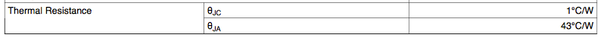Two thermal resistances are listed. θJC is the resistance from the device junction to the case. θJA is the thermal resistance from junction to ambient air. The latter is only relevant if we were to operate the LM3886 without a heat sink. So: θJC = 1.0 °C/W.

As the package of the IC is connected to the negative supply voltage, a thermal washer or pad is needed between the heat sink and the IC. These washers are designed to provide reasonable thermal conductivity while maintaining good electrical isolation. Traditionally, mica washers have been used in combination with thermal compound. If quality materials are used, this results in a thermal resistance of about 0.2~0.3 °C/W. The more popular (and certainly less messy) silicone washers have a thermal resistance of about 0.4 °C/W for the better ones. For exact numbers, see the manufacturer's data sheets. Note that in addition to the thermal washer, an isolating shoulder washer is needed as well. The ones intended for TO-220 packages work well.

For this example, let's use a silicone washer: θCS = 0.4 °C/W.

Finally, the junction temperature can be calculated: Tjunction = Tambient + Pdiss * (θJC + θCS + θSA) → Tjunction = 25 + 32 * (1.0 + 0.4 + 1.1) = 105 °C.

The observant readers will notice that this equation is of the same form as Ohm's Law. Temperature is analogous to voltage, power to current, and thermal resistance to electrical resistance. Ohm's Law, E = I × R, in the electrical domain, is analogous to T = P × θ in the thermal domain. This means we can simulate this thermal system using an electrical circuit simulator such as TINA-TI.Power is analogous to current, so Pdiss = 32 W becomes Idiss = 32 A. Temperatures become voltages, so the 25 °C ambient temperature is now a 25 V voltage source. The resistances just change units from °C/W or °K/W to Ω.

By plotting the node voltages in the TINA-TI simulation, the temperatures in the thermal system can be determined. We recognize the temperature of the heat sink (60.2 V = 60.2 °C) and the junction temperature (105 V = 105 °C).

Now let's see what happens if we use the isolated version of the LM3886 (or LM3886TF to be exact).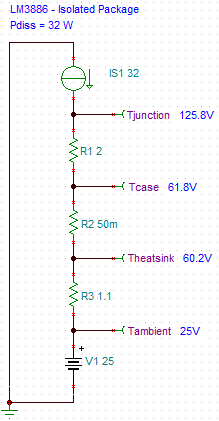With the isolated package, all you need between the package and the heat sink is a thin coat of thermal grease. The purpose of the grease is to fill in the voids in the surface roughness. The thermal grease is actually not very thermally conductive, but it's a lot more conductive than air, hence, a good quality thermal grease applied according to the manufacturer's specifications can reach a thermal resistance as low as θCS = 0.05 °C/W.

Note that the heat sink remains at the same temperature as with the non-isolated LM3886. This is expected, as the dissipated power is the same and the heat sink hasn't changed. However, note that the junction temperature is significantly higher at 126 °C. This is caused by the higher thermal resistance from junction to case, θJC. This thermal resistance is actually not specified in the LM3886 data sheet, but in Application Note AN-1192 (also known as BPA200), it is measured to be about 2 °C/W.

How about a stereo amplifiers with two LM3886 on the same heat sink? Still assuming ±25 V rails and 4 Ω load, the power dissipated in the heat sink is now twice as high, hence, to maintain the same heat sink temperature, the thermal resistance of the heat sink must be reduced by half, θSA = 0.55 °C/W. The simulation schematic is as follows: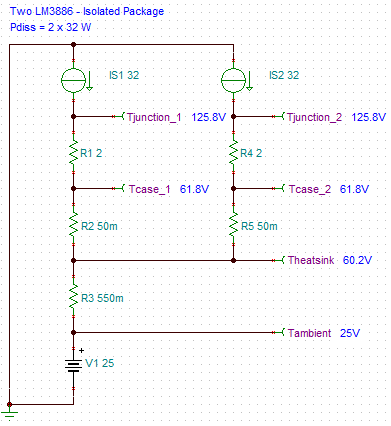A heat sink with a thermal resistance of 0.55 °C/W at ΔT = 35 °C would have to be specified for 0.55/1.257 = 0.44 °C/W at ΔT = 75 °C. 0.44 °C/W is a rather large heat sink. I have some older Wakefield heat sinks specified to be 0.4 °C/W. They measure 300 x 140 x 40 mm. The base thickness is 10 mm and the fin pitch is 10 mm as well. They weigh in at 2.1 kg each.

Most DIY enthusiasts seem to look at the headline specs and aim for those. Let's see what happens if we use ±35 V rails, use a 4 Ω load, and crank it to eleven. At an output power of 50 W, we find the highest power dissipation in the LM3886 to be 65 W. Then the thermals look like this:The heat sink reaches 77 °C. This is clearly not safe for an external heat sink! In addition, the junction temperature exceeds the ABSMAX by 60 °C. The amplifier will reach the thermal shutdown limit quickly and the SPiKe protection engage at even moderate output power levels. This is not a recipe for success or good sound. Thermal design needs to be done using a calculator rather than by guesswork and assumptions.

For more detail on heat sinks, semiconductor packages, thermal interfaces, and mounting techniques than you ever wanted to know, I suggest reading ON Semiconductor's Application Note, AN1040/D: "Mounting Considerations for Power Semiconductors".

Thermal Design Example 2: The Crest Factor

As some people will quickly point out, The Engineering Solution above results in a rather large, heavy, and expensive heat sink. They will also point out that most audio amplifiers are used for amplifying music signals, which are rather different from pure sine waves. These are perfectly valid points.

The main difference between music signals and pure sine waves lies in the crest factor. This factor describes the ratio between the peak amplitude and the RMS value of a signal:The crest factor is often expressed in dB:The crest factor of a sine wave is 1.41 (3 dB). The crest factor of music ranges from about 1.8~2 (5-6 dB) for heavily compressed heavy metal to approximately 10 (20 dB) for well-recorded classical music. This implies that an amplifier delivering, say, 100 W peak playing well-recorded classical music, only delivers about 1 W RMS. The same amplifier would deliver 50 W when reproducing a sine wave at the same peak power. There is a world of difference between the thermal design of an amplifier designed for 1 W RMS and one designed for 50 W RMS.

The burning question is then: Which crest factor should I design for? For an amplifier used in a home stereo to mostly play background music with the occasional use for a loud-ish party, designing for a crest factor around 10-20 dB is probably realistic. For example, Sound-on-Sound Magazine analyzed the crest factor of 4500 tracks of various types of music. The article is available on-line: "SOS: Dynamic Range & The Loudness War". Their sample collection had an average crest factor of 14 dB. I will use this number in the design example below.

To perform the thermal design, we need to calculate the amount of power dissipated in the LM3886 for a given output voltage swing. This requires a bit of arithmetic. You can find the full derivation in textbooks such as Sedra/Smith. I've summarized the highlights below. It is assumed that the amplifier is driven by a sine wave signal. The RMS power dissipated in the load can then be calculated as: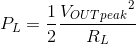PL is the power dissipated in the load, RL is the load resistance, and VOUTpeak is the peak output voltage.

Neglecting the idle dissipation for a minute, the power drawn from the power supply by the output stage can be calculated as:Where PS is the total power supplied by the power supply and PS+ and PS- are the powers drawn on the VCC and VEE supplies, respectively. It is assumed that a symmetric power supply is used, i.e. VCC = -VEE. Thankfully, this is normally the case.

The amount of power dissipated in the output stage is the difference between the supplied power and the power delivered to the load, i.e.,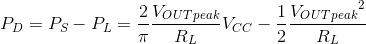where PD is the power dissipated in the output stage.

As shown above the dissipated power, PD, is a function of the peak voltage of the output swing, VOUTpeak. The peak output voltage at the maximum power dissipation can be found by taking the derivative of PD(VOUTpeak). I will spare you the arithmetic and provide the result: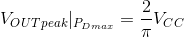Plugging this value into the equation for PD above, yields the peak power dissipation: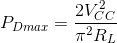Including the idle dissipation, the peak dissipated power works out to:where Ibias is the quiescent current of the output stage. As a sanity check, let's double-check the numbers from Example 1 above, where RL = 4 Ω, Vsupply = ±25 V, and Ibias = 50 mA (typical spec.). The peak power dissipation can be calculated as:This matches the 32 W read from Figure 35 in the data sheet rather well. The engineers at National Semiconductor may have forgotten to include the idle dissipation, which explains the 2.2 W difference between the calculated number and the one read from the graph.

Now, back to the crest factor... Using the load impedance and supply voltage from Example 1 (RL = 4 Ω, Vsupply = ±25 V), let's design the heat sink for a 14 dB crest factor. First the peak output voltage just before clipping is calculated: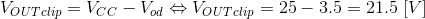At first glance, Vod is found in the spec table of the data sheet: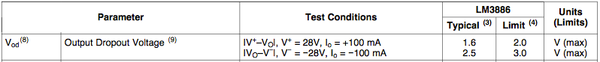However, note that the output dropout voltage is tested with a load current of 100 mA. To find the dropout voltage at more realistic load currents, we need to dig a bit deeper into the data sheet.Data sheet figures 14 and 15 show the dropout voltage for 4 Ω and 8 Ω loads, respectively. There are a couple of noteworthy details here. First off, note that Vod is strongly dependent on the load current, hence, considerably higher when the LM3886 is driving a 4 Ω than an 8 Ω load. Also, note the asymmetrical clipping, i.e. higher Vod when the LM3886 output voltage approaches the negative (VEE) rail than when it approaches the positive (VCC) rail.

The maximum swing without clipping is calculated using Vod = 3.5 V. The resulting peak output power, i.e. the instantaneous power at the crest of the sine wave, can be calculated as: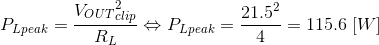From the peak power, the RMS power as function of the crest factor in dB can be calculated as: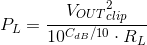It follows that the peak output voltage at an RMS output power of PL can be calculated as:The power dissipated in the LM3886 as function of crest factor may then be calculated from this equation: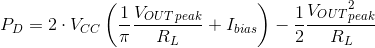This time, the quiescent current (or bias current) has been included in the equation. The dissipated power, including the quiescent dissipation, for a few commonly used crest factors is tabulated below.

Crest Factor (dB) PL RMS PL peak VCC RL PD
3 (sine wave) 58.0 W 116 W ±25 V 4 Ω 30.2 W
6 (max. PD) 29.0 W 116 W ±25 V 4 Ω 34.2 W
10 11.6 W 116 W ±25 V 4 Ω 29.2 W
14 4.60 W 116 W ±25 V 4 Ω 22.0 W
20 1.16 W 116 W ±25 V 4 Ω 13.4 W

As shown in the table above, if the amplifier is expected to play music with a relatively high crest factor, the size of the heat sink can be reduced dramatically, as considerably less power is dissipated under these conditions. This may complicate performance testing using sine wave signals, as the amplifier will overheat if driven continuously at the peak power dissipation if designed to a crest factor above 3 dB. However, the savings in heat sink weight, bulk, and cost usually outweigh engineering concerns about sine wave testing.

Thermal Design Example 3: The Duty Cycle

An approach similar to using the crest factor is to take advantage of the fact that the amplifier does not dissipate the peak power at all times. Rather, the amplifier is assumed to be operated at peak power dissipation only for some fraction of the time. This fraction is called the duty cycle, D = ton / (ton + toff), where ton and toff are the on-time and off-time respectively. In professional audio, a duty cycle of 33 % is commonly assumed.

Using the amplifier specs from Example 2 above (Vsupply = ±25 V, RL = 4 Ω, PDmax = 34.2 W) and a duty cycle of D = 0.33 (33 %), the average dissipated power can be calculated as: PDavg. = D * PDmax → PDavg. = 0.33 * 34.2 = 11.3 W.

For a more conservative estimate, designing for a duty cycle of 50 % might be a more reasonable goal: PDavg. = 0.50 * 34.2 = 17.1 W.

Even with the more conservative 50 % duty cycle, the savings in heat sink size are considerable.

Thermal Design Example 4: Other Solutions

If you have read this far, you have probably realized that my design philosophy is based in solid engineering and science. This approach is the most likely to produce products that will meet or beat the data sheet performance of the parts. By now you may have looked at some heat sinks and concluded, "OMG! THOSE THINGS ARE HUGE!!". For many DIYers, this reaction is typically followed by denial, "Joe Schmo on The Internet said his amp worked just fine with this itty-bitty heat sink. See! He has pictures of it. Physics must be wrong!". Sadly, however, physics is right. Mr. Schmo's amplifier probably works just fine at normal listening levels where the power dissipation in the LM3886 is barely above the idle dissipation. It may even work well at somewhat high listening levels, as, for most speakers in typical residential settings, this requires less than 1 W delivered to the speakers. Under these conditions, Mr. Schmo's amplifier probably works. Probably. So... Where's the limit? Given the finite limitations set by physics, what does the design continuum look like and which tradeoffs can be made to reduce the size of the heat sink?

As I see it, there are five reasonable options:

1. Reduce the rail voltage, thereby limiting the peak power dissipation.
2. Increase the load resistance, i.e. use an 8 Ω speaker rather than a 4 Ω one.
3. Use forced air cooling.
4. Add a thermal switch on the heat sink to turn the amplifier off before it overheats.
5. Design for the needed output power rather than the data sheet headline figures.

Reducing the rail voltage will result in an amplifier with a lower max. power output spec. This may not be palatable to some. Increasing the speaker impedance is also not likely to work for most people. Fans tend to be noisy, or require fan controllers to keep them quiet when the demand for cooling is low. As a result, most readers will likely look at options 4 or 5.

Regardless of how you plan to work around the limitations set by the laws of physics, make sure to do the math to ensure that the implemented amplifier can survive if someone decides to crank the volume knob and run the amp at high power output levels for a while. It may not be able to deliver the high output power indefinitely, but it should at least not self-destruct or endanger the user.

Thermal Cut-Off Switch

For an internal heat sink, the thermal protection circuit in the LM3886 is likely sufficient to protect the IC from melt-down, however, the heat sink and chassis may still get uncomfortably hot under these conditions. For an external heat sink, the heat sink temperature should be limited to 60 ºC for reasons outlined in Example 1 above. If you are skimping on the size of the heat sink, even if using the methods outlined in Example 2 and 3, I strongly recommend fitting the heat sink with a thermal cut-off switch. Such switches range from simple bi-metal switches, through thermistors, to semiconductor temperature sensors, such as the LM35. Regardless of the method of temperature sensing, make sure that once the thermal limit is reached, the amplifier turns off and remains off until turned on by the user. A little hysteresis, requiring the amplifier to cool down by at least 10 ºC before being allowed to be turned back on, would be a nice touch.

Real World Design Examples

A frequently asked question is, "how much power do I need?" Well. It depends, but maybe not as much as you think. For a more detailed answer, a little math is needed. I will work through the math using my home setup as an example. I encourage the reader to go through the math using the design variables for their setup. It's an eye-opening experience. For a while, my test speakers were KRK R6 passive studio monitors with a sensitivity of 87 dB (1 W, 1 m). This is a fairly typical sensitivity for a bookshelf speaker. My listening position was 180 cm (6 feet) away from the speakers. When I really cranked it and played, what I considered to be, loud music, the sound pressure level at the listening position measured about 85~90 dB(C). I measured using the SPL Meter App from Studio Six Digital.

This leads to the resulting design parameters: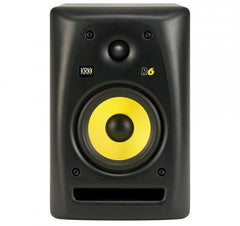Speaker reference SPL (sensitivity): SPLref = 87 dB

Speaker reference power: Pref = 1 W

Speaker reference distance: Dref = 1 m

Distance to the listening position:

Dlisten = 1.8 m SPL at the listening position:

SPLlisten = 90 dB

Number of speakers: N = 2

These days, my main speakers are KEF R700 with a sensitivity of 89 dB (1 W, 1 m), which is fairly representative of the sensitivity for a floor-stander. My listening position is 3 m (~10 feet) away from the speakers. 85~90 dB(C) SPL is still about what I use when I crank it. This leads to these design parameters: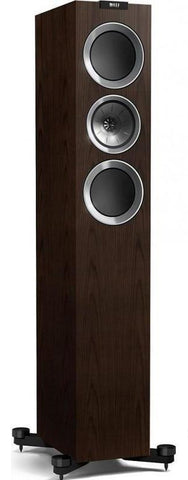Speaker reference SPL (sensitivity): SPLref = 89 dB

Speaker reference power: Pref = 1 W

Speaker reference distance: Dref = 1 m

Distance to the listening position: Dlisten = 3.0 m

SPL at the listening position: SPLlisten = 90 dB

Number of speakers: N = 2

Note that the KEF R700 is an 8 Ω (nominal) speaker.

To account for the larger listening distance, the attenuation as function of distance needs to be recalculated: Adist = 20 * log(Dlisten / Dref) → Adist = 20 * log(3.0/1.0) → Adist = 9.5 dB. Now, the applied power needed is calculated as, Pspeaker = Pref * 10(SPLspeaker + Adist - SPLref)/10 → Pspeaker = 1 * 10(87 + 9.5 - 89)/10 →Pspeaker = 5.62 W per speaker.

Similarly, the peak output power needed should be recalculated:

Ppeak = PRMS * 1014/10 → Ppeak = 5.62 * 1014/10 → Ppeak = 141 W.

The minimum supply voltage required to deliver this peak power can then be calculated as, VCC = √(Ppeak * Rload) + Vod → VCC = √(141 * 8) + 2.75 = 36.3 V.

Rounding off to ±36 V rail voltage, the power dissipation in the LM3886 works out to 22.7 W when delivering 5.62 W RMS to an 8 Ω speaker (calculated from the math above). 22.7 W is a bit easier to manage, thermally, than the 36 W that sine wave operation would dissipate, though, in practice quite a bit of heat sink is required even for 22.7 W of power dissipation.

Conclusion

Those who use their LM3886-based amplifiers in a desktop system or in smaller listening rooms will likely find that they can design for a much lower power output than initially thought. This will reduce the amount of heat sinking needed. However, those who use medium efficiency speakers in a larger room are likely better off designing for the highest power supply voltage the LM3886 will allow. As I note in the Output Power section, this means designing for a supply voltage of ±28-30 V if the amplifier is intended to power 4 Ω loads and ±35-36 V for 8 Ω loads. Designing for a 14 dB crest factor, this results in heat sinks of 1.4 K/W and 1.7 K/W for 4 Ω (±30 V) and 8 Ω (±36 V), respectively. If you insist on using smaller heat sinks, I strongly encourage you to fit them with a thermal cutoff switch.

Section 6: Power Supply Design